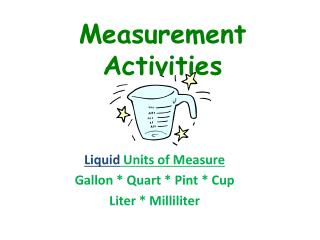DownloadDownload PresentationMeasurement Activities

# Measurement Activities

Télécharger la présentation## Measurement Activities

- - - - - - - - - - - - - - - - - - - - - - - - - - - E N D - - - - - - - - - - - - - - - - - - - - - - - - - - -
##### Presentation Transcript

1. Measurement Activities Liquid Units of Measure Gallon * Quart * Pint * Cup Liter * Milliliter

2. Estimate conversion between U.S. Customary and metric units, using ballpark comparisons, such as 1 quart is a little less than 1 liter 1 liter is a little more than 1 quart Quarts and Liters are just about the same! 7 liters is about 7 quarts 5 liters is about 5 quarts 81 liters is about 81 quarts

3. Equivalent Measurementsvolume US Customary System • cups & pints • pints & quarts • quarts & gallons Metric • milliliters and liters

4. Conversions Between Gallons & Quarts Q Q Q Q

5. Q Q Q Q FOUR QUARTS is the same as ONE GALLON

6. Three quarts is equivalent to _________ gallons

7. One quart is equivalent to _____ gallons

8. Two quarts is equivalent to _________ gallons

9. Conversions Between Gallons and Quarts FOUR QUARTS is the same as ONE GALLON Write the letter Q for every QUART that you have. Every time you have 4 of them, write a G around those letters to show that you have a gallon. Q Q Q Q

10. Sixteen quarts is equivalent to _________ gallons

11. Q Q Q Q

12. Write the letter Q for every QUART that you have. Every time you have 4 of them, write a G around those letters to show that you have a gallon. Q Q Q Q Q Q Q Q Sixteen quarts is the same as ____ gallons 16 quarts = 4 gallons Q Q Q Q Q Q Q Q

13. Twelve quarts is equivalent to _________ gallons

14. Write the letter Q for every QUART that you have. Every time you have 4 of them, write a G around those letters to show that you have a gallon. Q Q Q Q Q Q Q Q Twelve quarts is equivalent to _________ gallons 12 quart = 3 gallons Q Q Q Q

15. Conversions Between Pints & Cups C C

16. Conversions Between Pints & Cups TWO CUPS is the same as one PINT C C Write the letter C for every cup that you have. Every time you have two of them, write a P around those letters to show that you have a pint.

17. 5 cups = 2 ½ pints Half a Pint

18. 5 cups = 2 ½ pints Half a Pint This is 1 of the 2 cups needed to make another pint ….half a pint. C C C C C

19. 6 pints = 12 cups Write the letter P for each PINT C C C C C C Write the letter C Twice inside each P to show that TWO CUPS is the same as one PINT C C C C C C

20. 3 ½ pints = 7 cups Half a Pint 1 cup….half a pint. Write the letter P for every whole pint you have. C C C C C C Write two cups inside each pint to show that TWO CUPS is the same as one PINT C

21. Conversions Between Quarts and Pints PP

22. 3 quarts = 6 pints Write the letter Q for every QUART you have. Write two PINTS inside each QUART to show that TWO PINTS is the same as one QUART PP PP PP

23. 11 pints = 5 ½ quarts

24. 11 pints = 5 ½ quarts Every time you have 2 PINTS, write a Q around those letters to show that you have a quart. Write the letter P for every PINT you have. One PINT makes.half a quart. PP PP PP PP PP P

25. Conversions Between Liters & Milliliters Liters x 1,000 - Milliliters This Pattern is EASY to see in a chart.

26. Can you complete the chart?

27. Try these… • Use the chart to solve: • 3 gal. = _________ quarts • 5 pints = _________ cups • 7 quarts = _________pints • 3 liters = ________ml • 8 liters = _________ ml • 15 liters = ________ ml